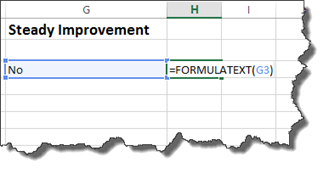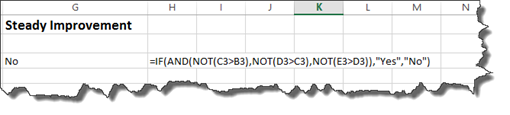Understanding Data Lookups in Excel 2016

Data lookup is quite simply the process where values in Excel are scanned until certain results are found.   In Excel 2016, there are two main formulas for looking up the data you have in a worksheet.  There is VLookup where the V stands for vertical, and there is HLookup where the H stands for Horizontal.

## The Basic VLookup Formula

Below is the basic VLookup formula.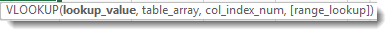Inside the brackets, you will see the information that Excel asks us to enter into the formula.

You can also go to the Formulas tab, click the Lookup & Reference button, then select VLookup.

You will then see this dialogue box: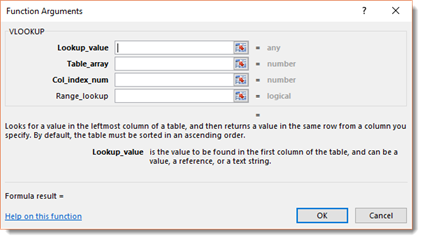The benefit of using the dialogue box when entering a formula is the bit of instruction that Excel gives you for each field.   If you are newer to Excel, it is recommended that you use the Function Arguments dialogue box to create your formulas.   It is a lot less trial and error that way.

For the time being, however, let's look the formula in our worksheet again.• Lookup_value is the value that we want to look up.

• The table_array is the table where the data will be retrieved. This value can be a range or the name of a range.

• Col-index_num is the column number in the table. The first column in your table is column 1.

• Range_Lookup is typically true for false. True will give you a near match. If you want to find an exact match, it is false.

Let's learn how to use the VLookup formula.

Take a look at our worksheet below.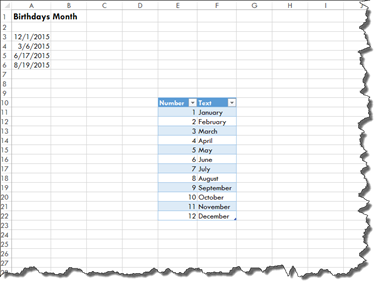On the left, we have birthdays listed. We know that 12 means December, and we could use the MONTH function to fill in December for us, but instead we want the text value.

On the right, we have created a lookup table. We are going to use a VLookup to find the text value.

We start by entering the VLOOKUP function, then open brackets.

The first piece of information we must supply is the lookup value. We know the lookup value is the month located in Column A (the first column), but we must use the MONTH function to specify this, as shown below.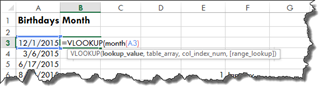Next, we add a comma, then for the table_array, we are going to highlight the cells in the table; however, we do not highlight the column headers.

Enter another comma.

Now, for the col_index_num.   Ours is Column 2. We then enter another comma.

Since we want the exact match, we enter FALSE.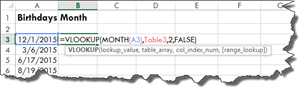Close the brackets, then push Enter.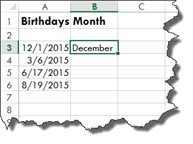A lookup table is created by simply creating a table in Excel.   It is used as a master list of sorts, and you will use it to locate data that you need to find using search criteria that you will enter into your lookup formula.  You can use a huge table of data that you have created as your lookup table. You do not have to create a lookup table just to use either the VLookup or HLookup functions.

For example, let's say you have a table within a spreadsheet that contains 15,000 customer names, complete with address, billing information, shipping information, anniversary dates, and etc.   You can use a lookup formula to find specific data within the table. If you wanted to find the address, for example, you could create a lookup formula to find that within your table of data.

## Naming Lookup Table Data

If you are looking up data that may be on different worksheets within a workbook, it makes it easier if you name the data you are going to be searching.

This is easy to do.

Go to the lookup table data. Click anywhere in the data, then press CTRL+A. This selects all of the data.

Next, go to the Formula tab.

Click the Define Name button in the Defined Names group.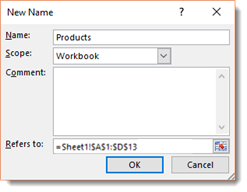Enter a name for the group.    The scope is workbook.

Click OK.

Now, when you enter the table_array information into your data lookup, you can simply enter this name. In our case, it is products. You will see it appear in a dropdown menu.

Please note that if you add data to the end of a lookup table after you have named it, then enter the name of the data into Excel (we named ours Products), the formula will not retrieve that data because it is not part of your named data.

We entered another product to our product list below.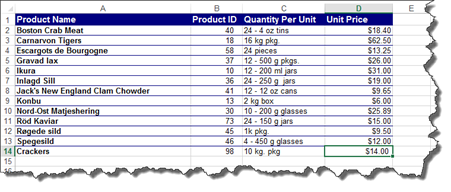Now we can go to our Name box, and choose the name of your data.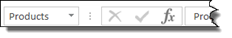As you can see, the last row is not included.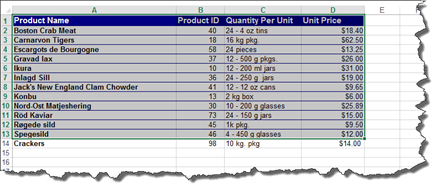If you add rows or columns to a lookup table after you have named it, insert the data into the existing data table. Do not put it at the very beginning or the very end. Otherwise, you will have to rename it, then change your lookup formula.

Look what happens when we insert the new row above our last row.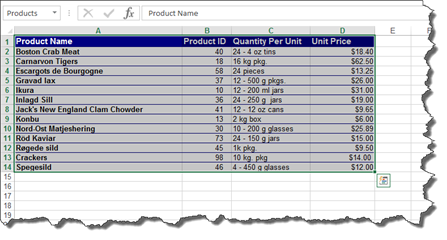It is now included with the data.

However, you can always add extra blank rows in your lookup table so that you have room to add new things at the end.

## VLOOKUP: A Real Example

It is important that you understand exactly how VLOOKUP works. For that reason, we are going to show a more realistic example.

Below we have an Excel invoice. This is just one of the pre-built templates that we are going to use.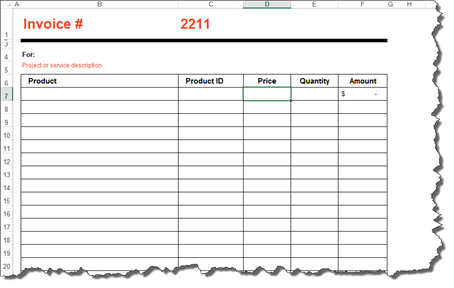We also have a product list in another worksheet.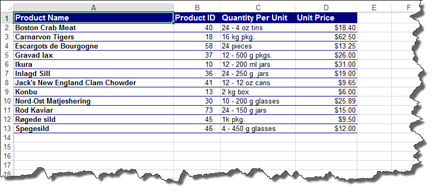We are going to use VLOOKUP to find the product ID and the price per unit for our invoice.

Take a look at our invoice again.

We have entered in Crab Meat as the first product sold.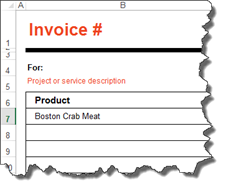Now, we are going to use VLOOKUP to find the product ID.

Enter your VLookup formula in the cell for the product ID.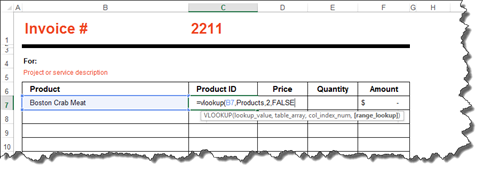Notice that we entered in Products for the table_array.

Hit Enter.

You should now have the product ID in the cell.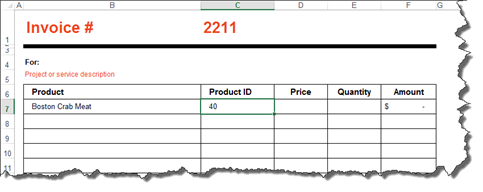Now let's do the price.

As we fill in our formula, notice that when we get to table_array and type in the name for our data, it appears in a dropdown list.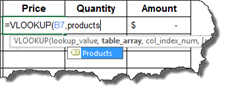Finish entering in the formula.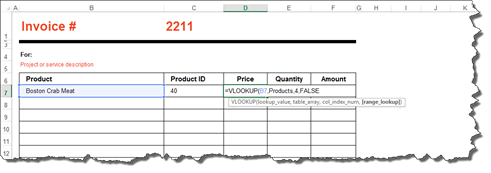Close the brackets when you are finished entering the formula, then press Enter.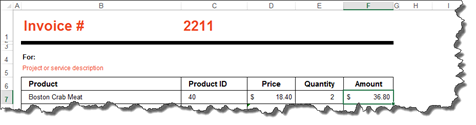Whereas VLOOKUP is vertical lookup, HLOOKUP is horizontal lookup. However, vertical or horizontal lookups do not refer to the table in which you want to place the results. Instead, it refers to the table in which you are using to search for data.

When you use the HLOOKUP function, you are looking for data horizontally. In VLOOKUP, we looked in a column for data. Columns are vertical. In HLOOKUP, you search rows. Instead of entering the column number, you will enter the row number, as shown below.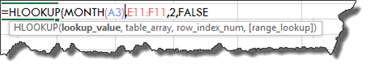Add the end bracket to the formula, then press Enter.

## Working with Near Matches

Let's talk about range lookup in the VLOOKUP and HLOOKUP formulas.   As we already have learned, if you enter FALSE as the range lookup, Excel will return the exact match for the results.

However, if you use the TRUE argument for a lookup, it will use the nearest match that is of the next largest value if an exact match is not found.

For our lookup table, we have a grading scale.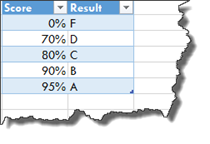We are going to use VLOOKUP to input the letter grade.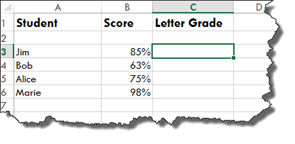Below is our formula. Notice that we named our data "score."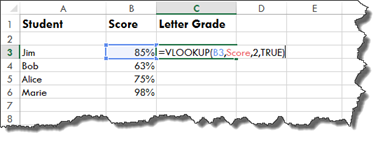Hit Enter.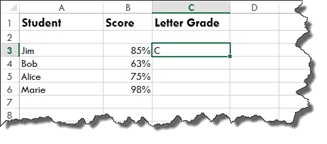Now, drag the handle in the lower right hand corner of the results cell to fill in the rest of the data.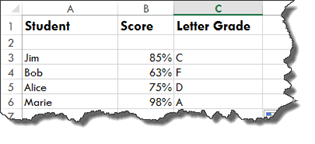## When There is Missing Data in a Lookup

If you use VLOOKUP or HLOOKUP, and Excel cannot find the data you are looking for, you will see this message appear in the cell after you press Enter to see results.

Interested in learning more? Why not take an online Advanced Excel 2016 course?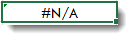What you will want to do if you see this message is to run an IF statement to make sure the data really is not there.

Let's use our invoice and product list as an example. We are going to create the formula, product the #N/A message, then run the IF statement.

In our invoice below, we have entered a product that we know does not exist in our product list.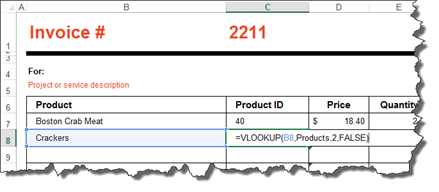We are going to hit Enter to see the results of the formula.

As we already knew would happen, Excel can't find the results.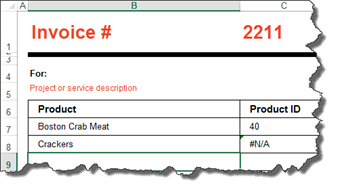To make sure the lookup is correct, we are going to modify our formula so we can run an IF statement.

To start with, we typed IF after the = for the IF statement, then we entered an opening bracket and typed "isna."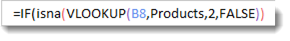We also added a closing bracket to the end to close the IF statement and our VLOOKUP. As you can see above, our VLOOKUP formula sits inside the "isna" formula.

If the result really is NA, we want Excel to give us 0 as a result because there is not a product number.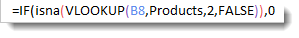If there is a value, we want it to do a VLOOKUP again, so we copy and paste the VLOOKUP in after placing another comma.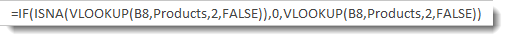As you can see, we then get a zero because the data is indeed missing.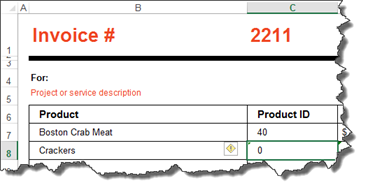## Nesting a Lookup Within a Lookup

You can nest a lookup within a lookup to go through multiple tables and pull out a value.

For example, in our worksheet shown below, we have a list of employees with the years that they have worked for our fictional company.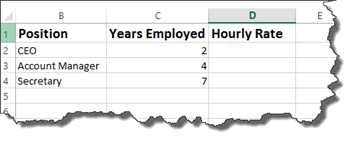We want to use our lookup tables to determine their hourly rate of pay.

That said, our fictional company determines an employee's hourly pay by their job classification, then the number of years they have been on the job.

We have two data tables for that.

Here is the first. We named it "class."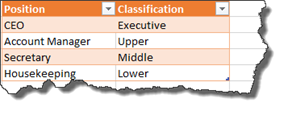As you can see, this table lists the various job positions in the company, then lists the classification for each position.

Here is our second table. We named it "salary."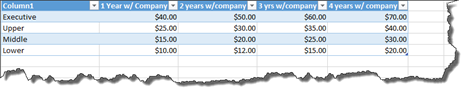This table lists the different classifications, then the hourly rate of pay for those classifications based on the number of years the employee has been on the job.

To figure out the hourly rate for our worksheet, we will use nested VLOOKUPS.

Let's start out by using VLOOKUP to find the job classification for the first employee in our list.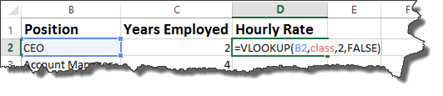Now we will nest this VLOOKUP inside another VLOOKUP.

To do this, we will put the cursor after the equal sign in our formula, then type VLOOKUP again, followed by an opening bracket.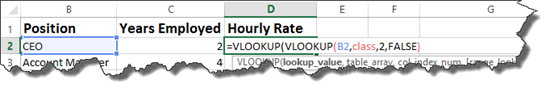Put a comma after the closing bracket, then enter your table array for the new VLOOKUP, the column number, then FALSE for an exact match – as shown below.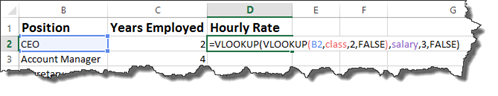Hit Enter.

Our formula should now show the hourly rate for the employee.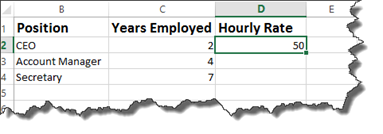If we consult with the tables, we see that \$50.00 is indeed the hourly rate for a CEO who has been with the company for two years.

Now we can figure out the hourly rate for the rest of the employees.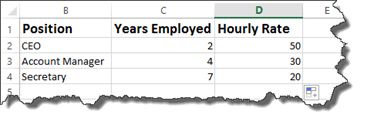## The IF Function

The IF function helps to determine what will be displayed to those who view your worksheets in Excel. Because of its purpose, it is one of the most important functions you will learn. IF functions can be used to add comments to your data. They can also be used to hide errors in calculations.

The syntax for the IF function is =IF. This lets Excel know that it is an IF function.

Then, we begin the formula that Excel will use to produce the results we want.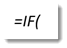Next, we add the evaluation criteria. For example, is six greater than three? The evaluation criteria asks a question. The question has two outcomes. If the answer (or outcome) is correct, such as "Yes, six is greater than three," then the answer is true. If not, it is false.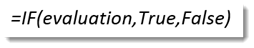If it is true, we put what happens when the answer is true.

If it is false, we put what happens when it is false.

In the worksheet below, we have a list of employees, followed by the rating they received as part of their annual review.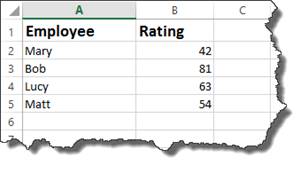Now, we want to add another column called Comments.   In this column, we want to add comments about the scores. This way, when a manager looks at the worksheet, he/she does not need to worry themselves with the actual rating – and what that means. They can simply look in comments.

To add a comment based on the rating, we are going to use the IF function.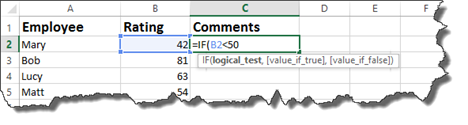We have started the formula in the snapshot above.

We have said if the employee's rating is less than fifty…

Now we enter what happens.

If it is correct that the employee's rating is less than fifty, the comment should be "needs significant improvement.   However, if it is false and if the rating is not less than fifty, the comment should be needs improvement.

Notice that we use quotation marks to enter the comments into the formula.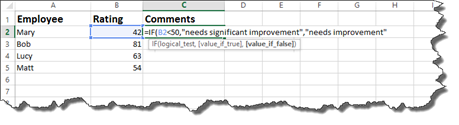Now, add an end bracket, then push Enter.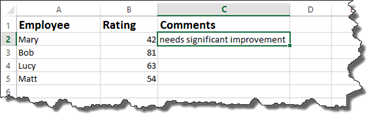Since this contains a formula where all are cell references are relative, you can use the handle in the lower right corner of the cell, then drag it down to complete the comments for the other employees.

You can also use the IF function to hide Excel error messages.

Let's show you what we mean by taking a look at the worksheet below.

In this worksheet, we have a formula that will divide cell C2 by cell D2.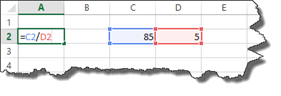If we push enter in cell A2, it will give us the answer.

However, let's say the data in cell D2 is missing.

When we push Enter, we see an error message.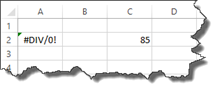We can use the IF function to hide this error message.

To do this, we write the formula below: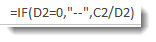Let's translate what it says.   If D2 equals 0, enter two dashes into cell A2 where we have our formula. If it does not equal zero, then go ahead and divide cell C2 by D2.

If we would put the number five back into cell D2 and leave the IF formula in place, we would see the answer to C2/D2.

Here is our worksheet below. We have hidden the error message with two dashes.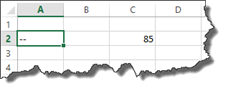NOTE: All of the IF functions appear under the Formulas tab and by clicking the Logical button. From there, you can access the Function Arguments dialogue box.

## The IFNA and IFERROR Functions

The IFNA function tells Excel what to do if an #NA error is produced, whereas the isna tells Excel what to do if the returned value is #NA.

The #NA error appears in a cell when a value is not available to a formula.

If in our worksheet below, we wanted to find the mode, we would enter this formula: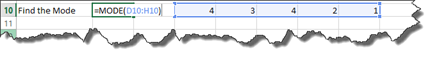When we push Enter, the result is displayed.However, if we went back and changed the second instance of the number four to a six, we would see an #NA error because the value needed is not available to determine the mode.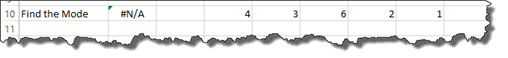To avoid #NA values from appearing, we could use the new IFNA function.

It would be entered like this: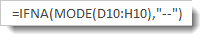All of the IF functions appear under the Formulas tab and by clicking the Logical button. The easiest way to use the IFNA function is to click the Logical button, then select IFNA.

Below you can see the dialogue box for the IFNA function.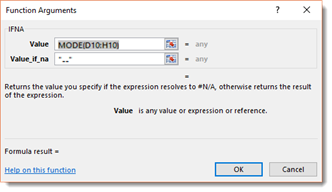The IFERROR function can be used as a general "cover all" for any errors that might appear in your data. You can use it to specify what will be entered if any error occurs in the calculation of a formula.

It is written the same way as an IF function. However, instead of writing IF, you would write IFERROR. You can access the dialogue box for IFERROR by going to the Logical button under the Formulas tab.

## Nesting IF Statements

Just as with VLOOKUPs and HLOOKUPs, you can nest an IF statement within an IF statement.

In our worksheet below, we have a list of friends, along with their ages.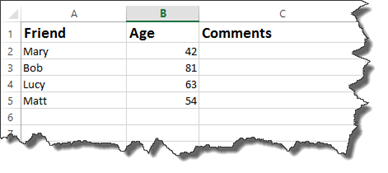We could create a simple IF formula to enter comments.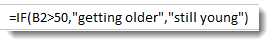This says if the age is greater than 50, then the comment is getting older. If it is less than 50, then they are still young.

If we nest an IF statement within the current IF statement, we would keep the evaluation criteria, but we would replace one of the outcomes with our new IF statement. However, keep in mind, then when you nest an IF statement, you do not need another equal sign.

Take a look at our new formula below: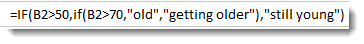We have our original IF statement intact. We have highlighted the first part of it below.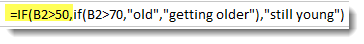If the age is greater than 50, followed by the two outcomes.

As you can see in our formula above, the TRUE outcome has another IF statement.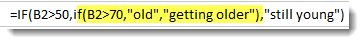The nested IF statement tells Excel that if the age is greater than 70, enter the comment "old." If it is not, enter getting older.

Our worksheet is shown below with the nested IF statements determining what comment is displayed.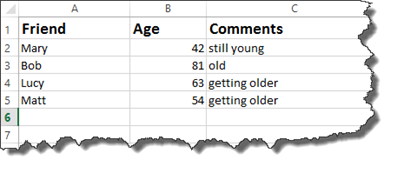Now, let's break this down into easy to understand terms.

When you enter the formula above with a nested IF statement, Excel reads the first IF statement. It looks at your criteria, then the outcomes for the criteria.

If the outcome for the first IF statement is true, Excel is then going to perform another IF statement to determine what appears in the comments.   It will put "old" if the age is greater than 70. It will put getting older if it is not greater than 70.   It is very important to remember that these two outcomes both serve as your TRUE outcome for the first IF function.

Now, if your original IF statement returns a FALSE outcome, then Excel will enter "still young."

A nested IF statement gives you a way to enter more than one response for an outcome.

We used a nested if statement for the TRUE outcome. However, you can also use a nested IF statement for a FALSE outcome.

If the nested IF statements are still confusing to you, remember it this way: the nested IF statement provides two additional outcomes for either the TRUE or FALSE outcome of your original IF statement.

## Using the AND Operator in an IF Statement

You use the AND operator within an IF statement if you have more than one criteria that needs to be true. Using the AND operator can sometimes be easier to write than multiple nested IF statements.

Take a look at the worksheet below.

In it, we have a list of students and their grades for the four grading periods.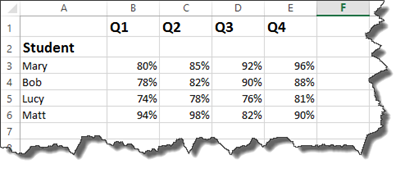Each student must have earned greater than 80% in each quarter in order to receive an award. We will use the AND operator to set this as criteria.

Take a look at how at the formula bar to see how we wrote this.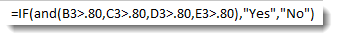Now, let's examine it.

We start out by starting the IF statement with =IF, then the open bracket.

Next, we write AND for the AND operator.   The criteria then is placed within brackets. Notice that we have four sets of criteria. The criteria is that each quarter's grades must be greater than 80%. Also notice that all percentages have been converted to decimals when entered in as criteria.

After you have entered the criteria in brackets, you can enter your TRUE and FALSE values.   We want the cell to display "Yes" if the criteria is met.   We want it do display "No" if it is not met. We then type in an end bracket to close the IF statement.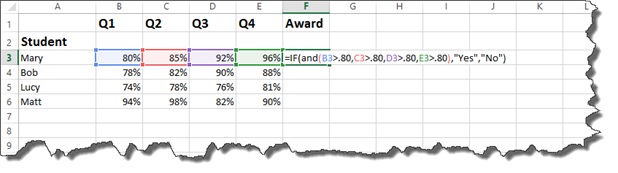Press Enter.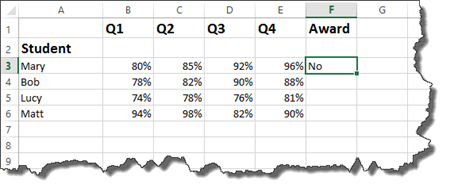As you can see above, student Mary had one grade that was 80%. All of the grades had to be above 80% to meet our criteria, so a NO was returned.

You can now drag the handle in the cell down to complete the worksheet for all students.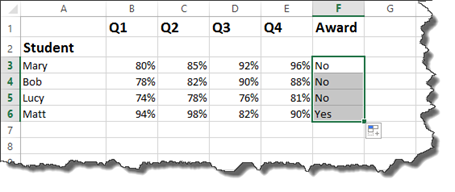## Using the OR Operator in an IF Statement

The OR operator works in the same way as the AND, except it says if one of the criteria listed is true, then the outcome for TRUE is what is displayed.

Let's look at our worksheet.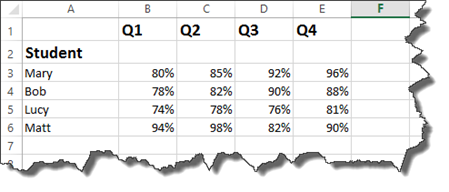This time, we want to say if the grade for Q1, Q2, Q3, or Q4 is greater than 90%, then the student gets the award.

You enter it in the same way you did with the IF statement and the AND operator, expect you type OR instead of AND.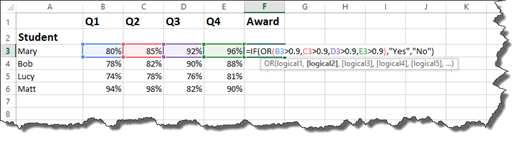Press Enter.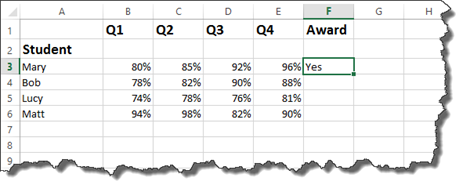You can then complete the worksheet.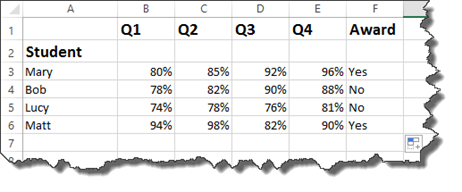Now let's make it a bit more complicated.

Now we want to say that in order to get the award, the average of all four quarters must be greater than 90% OR at least one of the quarters must be greater than 95%.

In other words, the average of the quarters must be greater than 90%. Or Q1, Q2, Q3, or Q4 must be higher than 95%.

This is the perfect time for you to test our your ability writing formulas by entering the formula into the cell.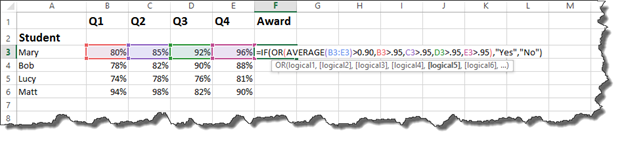Press Enter.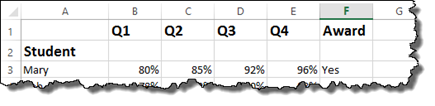## Using the NOT Operator

The NOT operator is used in conjunction with the AND or OR operator.

The best way to explain how the NOT operator works is to show you the NOT operator in action.

Working with our students and grades again, we want to determine if the grades have improved each quarter.

To do that, we are going to use the IF statement with the AND operator since we are using multiple criteria.

We will use the NOT operator to make sure Q1 is not higher than Q2. Q2 must be greater than Q1.

In addition, Q3 must be greater than Q2, and Q4 must be greater than Q3.

We have started the write it below: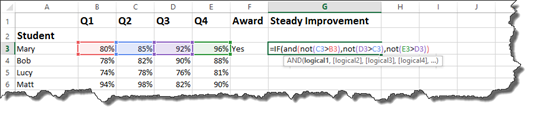Let's finish.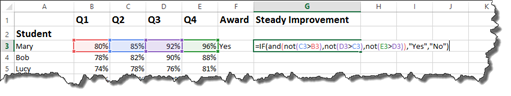Hit Enter.

We can see there is not steady improvement.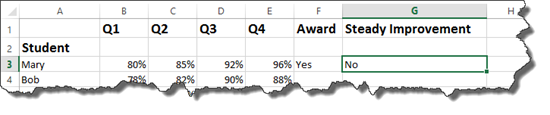Remember: The NOT Operator is used within AND or IF options. You must use the NOT operator for each piece of criteria. You cannot type in the NOT operator once, then use it for all criteria.

## Displaying Cell Formulas In Another Cell

Starting with Excel 2016, you can display the formula from one cell in another.   In our worksheets so far, we could view the formula in a cell by double clicking on the cell. However, once we pressed Enter or tabbed out of a cell, we could not see the formula unless we looked in the Formula Bar.

To display a formula from one cell in another cell, go to the cell where you want to formula to appear.

You will use the FORMULATEXT function.

Type the equal sign, followed by formula text.

Enter an open bracket, then click the cell that contains the formula you want to display.